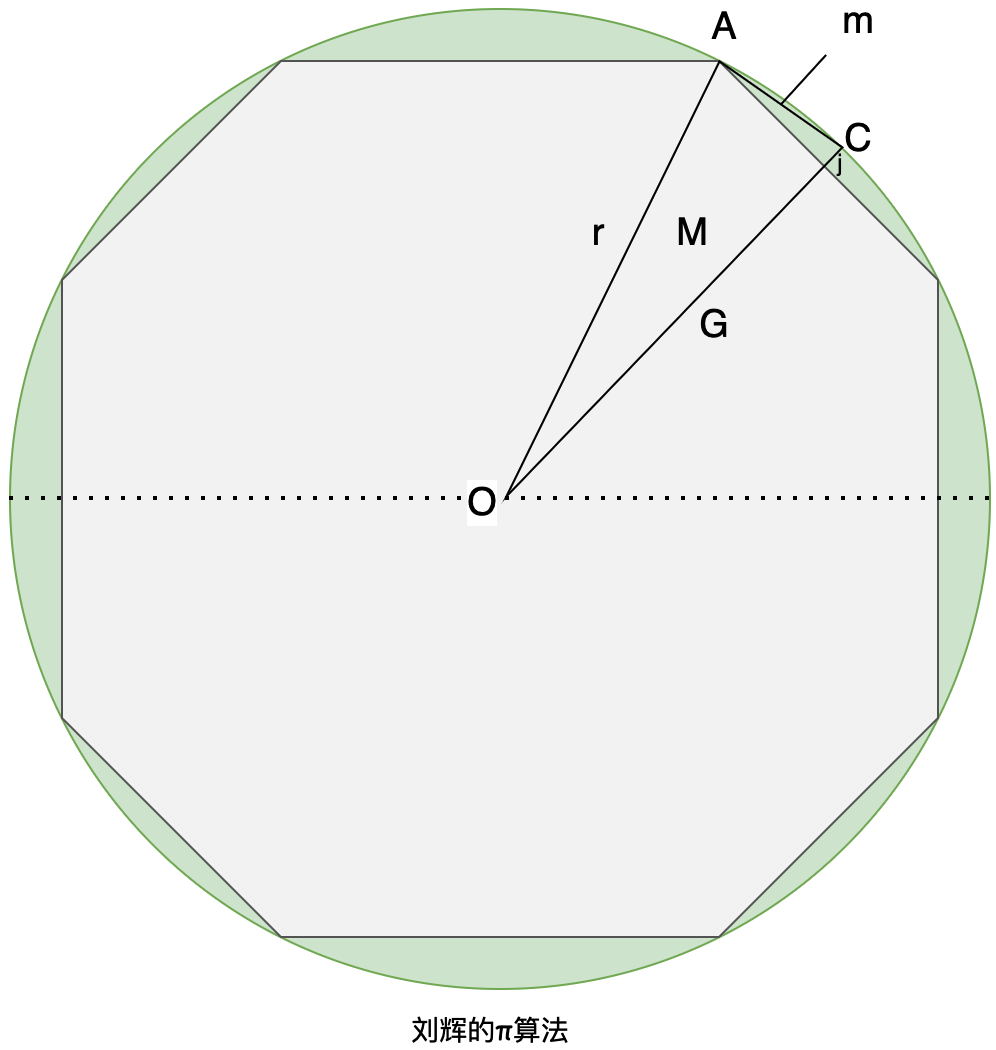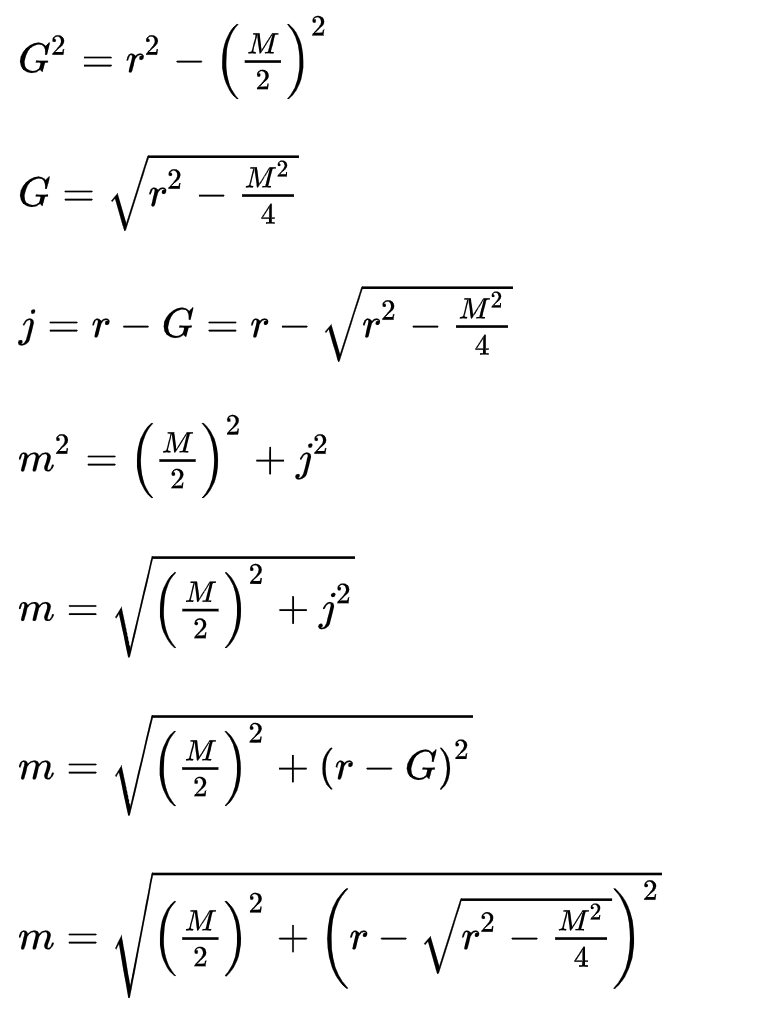# # 《程序员数学：割圆术》—— 基于 N-gons 的近似 π 计算

`割圆术的历史`

## # 二、刘辉算法`AB`用线平分`OPC``AC`成为十二边形（12 边形）的一侧，令其长度为`m`。让 be 的长度和be`PC``j`长度。`OP``G

`AOP`,`APC`是两个直角三角形。刘徽反复引用勾股定理 (opens new window)（勾股定理）：## # 三、算法实现

### # 1. 复杂度高

``````public static double liuHui01(int splitPoint) {
// 圆的半径
double r = 1.0;
// 正方形的边长
double s = 2.0 * r;
Random rand = new Random();
// 计算圆内随机生成的点的个数
int m = 0;
for (int i = 0; i < splitPoint; i++) {
double x = rand.nextDouble() * s - r;
double y = rand.nextDouble() * s - r;
if (x * x + y * y <= r * r) {
m++;
}
}
// 面积比 = 圆的面积 / 正方形的面积
double p = (double) m / splitPoint;
// 圆周率 = 面积比 * 4
return p * 4;
}
``````
1
2
3
4
5
6
7
8
9
10
11
12
13
14
15
16
17
18
19
20
• liuHui01使用了一个叫做重心法的算法，将圆划分成若干个小正方形，然后在每个小正方形内随机生成点，最后统计出有多少个点在圆内。
• 这个方法接收一个整数 splitPoint，表示圆内划分的小正方形的个数。它首先声明了一个叫做 r 的常量，表示圆的半径，然后计算出正方形的边长。然后，它创建了一个随机数生成器 rand，并循环 splitPoint 次，每次生成一个随机数对 (x, y)，表示在某个小正方形内的点的坐标。接着，它判断这个点是否在圆内，如果是，就将计数器 m 加 1。
• 最后，它计算出圆内的点的占比，并将这个占比乘以 4，得到圆周率的近似值。

### # 2. 复杂度低

``````static double getNGonSideLength(double sideLength, int splitCounter) {
if (splitCounter <= 0) {
return sideLength;
}
double halfSide = sideLength / 2;
// 使用勾股定理（勾股定理）
double perpendicular = Math.sqrt(Math.pow(circleRadius, 2) - Math.pow(halfSide, 2));
double splitSideLength = Math.sqrt(Math.pow(excessRadius, 2) + Math.pow(halfSide, 2));
return getNGonSideLength(splitSideLength, splitCounter - 1);
}

static int getNGonSideCount(int splitCount) {
// 内接六边形 (6-gon) 开始
int hexagonSidesCount = 6;
// 在每次拆分迭代中，我们制作 N 边形：6 边形、12 边形、24 边形、48 边形等等。
return hexagonSidesCount * (splitCount > 0 ? (int) Math.pow(2, splitCount) : 1);
}

public static double liuHui02(int splitCount) {
double nGonSideLength = getNGonSideLength(circleRadius, splitCount - 1);
int nGonSideCount = getNGonSideCount(splitCount - 1);
double nGonPerimeter = nGonSideLength * nGonSideCount;
double approximateCircleArea = (nGonPerimeter / 2) * circleRadius;
}
``````
1
2
3
4
5
6
7
8
9
10
11
12
13
14
15
16
17
18
19
20
21
22
23
24
25
26
• 方法 liuHui02 接收一个整数 splitCount，它表示圆内进行的拆分次数。在内部，这个方法调用了两个静态方法 getNGonSideLength 和 getNGonSideCount，分别用来计算所得多边形的边长和边数。然后，它使用这些值计算出多边形的周长，并利用这个周长来估算出圆的面积。最后，它返回圆的面积与多边形的面积之比。
• 静态方法 getNGonSideLength 递归地使用勾股定理来计算所得多边形的边长。这个方法的第一个参数是边长，第二个参数是拆分次数，在拆分次数不为正数时递归终止，并返回边长。否则，它会将边长分成两半，并使用勾股定理计算出所得多边形的新边长。然后，它会使用新边长继续进行递归，直到拆分次数变成正数为止。
• 静态方法 getNGonSideCount 使用与 splitCount 相关的算法来计算所得多边形的边数。在这个方法内，有一个常量 hexagonSidesCount 被初始化为 6，表示一个内接的 6 边形。然后，它会根据 splitCount 的值来进行计算。如果 splitCount 是正数，那么它会返回 hexagonSidesCount 乘以 2 的 splitCount 次方，否则就返回 hexagonSidesCount 本身。这个方法的返回值就是所得多边形的边数。
• 综上所述，这段代码通过计算内接的多边形的边长和边数，并使用这些信息估算圆的面积，最后计算圆的面积与多边形的面积之比。

https://en.wikipedia.org/wiki/Liu_Hui%27s_%CF%80_algorithm (opens new window)